# Oberseminar Analysis Winter 2017/18

## Organizers: S. Schwarzacher, S. Conti, H. Koch, S. Müller, B. Niethammer, M. Rumpf, C. Thiele, J.J.L. Velázquez

• Thursday, October 19, 2:15 p.m., Lecture hall 0.008
Richard Höfer (University of Bonn)
Particle sedimentation in Stokes flows
In this talk, we will consider the N-particle dynamics of spheres immersed in a fluid subject to gravitation and study the limit of many small particles. We will discuss the physical relevant quantities that determine the macroscopic behavior of the cloud of particles. A rigorous convergence result is presented for the inertialess dynamics. The limit equations resemble the equations for a Stokes fluid of variable density.

• Thursday, November 2, 2:15 p.m., Lipschitz-Saal
Filip Rindler (Warwick University)
Rademacher's Theorem, Cheeger's Conjecture and PDEs for measures
The classical Rademacher Theorem asserts that every Lipschitz function is differentiable almost everywhere with respect to Lebesgue measure. On the other hand, Preiss (’90) gave a surprising example of a nullset in the plane such that every Lipschitz function is differentiable at at least one point of this set. Thus, it is a natural question to ask whether there exists a singular measure such that all Lipschitz functions are differentiable with respect to this singular measure. It turns out that this question has an intricate connection to the geometric structure of normal one-currents. In this talk I will present a converse to Rademacher’s Theorem, which settles the question in the negative in all dimensions: if a positive measure μ has the property that all Lipschitz functions are μ-a.e. differentiable, then μ is absolutely continuous with respect to Lebesgue measure (in the plane, this question was already solved by Alberti, Csornyei and Preiss in ’05).
In a geometric context, Cheeger conjectured in ’99 that in all Lipschitz differentiability spaces (which are essentially Lipschitz manifolds in which Rademacher’s Theorem holds) likewise there is a “functional converse” to Rademacher’s Theorem. As the second main result, I will present a recent solution to this conjecture.
The proofs of both of these theorems are based on a recent structure result for the singular parts of PDE-constrained measures, its corollary on the structure of normal one-currents, and the powerful theory of Alberti representations.
This is a joint work with A. Marchese and G. De Philippis

• Thursday, November 23, 2:00 p.m. (s.t.), Lecture hall 2.040
Francesco Ghiraldin (University of Basel)
Some recent results on measures arising from variational problems
In this talk I will present some results on the structure of measures that are obtained from minimizing sequences in variational problems. The case study is the Plateau problem, namely the minimization of a Lagrangian among a class of surfaces encoding a certain notion of boundary condition, the simplest being the area functional. This family of problems has been described and formalized in several ways: After reviewing some established results, I will show how a-priori estimates obtained from energetic comparison easily lead, at least in the isotropic setting, to an existence result for a broad class of formulations of the problem. In the anisotropic setting, further challenges arise from the oscillation of the tangent planes as well as from possible concentration phenomena. In this case, using the machinery of varifolds, I will present a condition on the Lagrangian that is necessary and sufficient to obtain the expected tangent structure of the limits of minimizing sequences; as a consequence, lower semicontinuity and existence theorems follow. This last result holds for varifolds having bounded anisotropic first variation and therefore generalizes the celebrated rectifiability result obtained by Allard [On the First Variation of a Varifold, Ann. of Math., 1972].

• Thursday, November 30, 2:15 p.m. (s.t.), Lipschitz-Saal
Raphael Winter (University of Bonn)
From a non-Markovian system to the Landau equation
We consider the weak-coupling scaling limit, which models the evolution of particles in a plasma. An open problem in kinetic theory is to prove the convergence of the macroscopic evolution to a solution of the Landau equation. In this talk, we take as a starting point the (formal) leading order behavior of the system in the scaling limit, that can be derived under the assumption of propagation of chaos. We prove the well-posedness of this system on the macroscopic timescale, and the convergence to a solution of the Landau equation. The limit shows the transition from an evolution with memory effects and finite speed of propagation to a dissipative, parabolic limit.

• Thursday, December 7, 2:15 p.m., Lipschitz-Saal
On the splat singularity for the Muskat problem
This talk deals with different aspects of the Muskat problem. This problem studies the evolution of the interface between two immiscible fluids of different nature in a porous medium. We study two different regimes: homogeneous and inhomogeneous. For the homogeneous case, namely, the medium has constant permeability; the study of “splat” singularities for the one-phase Muskat problem is carried out (a single fluid in the vacuum is considered). For the inhomogeneous case, considering the permeability of the medium as a step function; a study of the local existence in Sobolev spaces and finite time singularities of “splash” and “splat” type is performed.

• Thursday, December 14, 2:15 p.m., Lipschitz-Saal
Christina Lienstromberg (University of Hannover)
On microelectromechanical systems with general permittivity profile
Qualitative properties of solutions to the evolution problem modelling microelectromechanical systems with general permittivity profile are investigated. The system couples a parabolic evolution problem for the displacement of a membrane with an elliptic free boundary value problem for the electrostatic potential in the region between the membrane and a rigid ground plate. Assuming to have a non-positive displacement, it is shown that the solution develops a singu- larity after a finite time of existence. Moreover, numerical evidence is provided that reveals substantial qualitative differences between the investigated coupled problem and the widely-used small-aspect ratio model.

• Thursday, December 14, 3:45 p.m., Lecture hall 2.040
Kathrin Welker (University of Trier)
Optimization in Shape Spaces
Shape optimization problems arise frequently in technological processes which are modelled by partial differential equations or variational inequalities. In many practical circumstances, the shape under investigation is parametrized by finitely many parameters. This allows the application of standard optimization approaches, but limits the space of reachable shapes unnecessarily. The differential-geometric structure of certain shape spaces is investigated and applied to the theory of shape optimization problems. Furthermore, the space of so-called H 1/2 -shapes is defined. The H 1/2 -shapes are a generalization of smooth shapes and arise naturally in shape optimization problems.

• Thursday, December 21, 2:15 p.m., Lipschitz-Saal
Zorin-Kranich Pavel (University of Bonn)
Maximal polynomial modulations of singular integrals
A maximally modulated singular integral operator first appeared in connection with the problem of pointwise convergence of Fourier series of L2 functions, resolved by Carleson. The question whether singular integrals maximally modulated by polynomial phases are bounded on Lp has been raised by Stein in connection with singular integrals on nilpotent groups. We answer this question positively for p larger than 2, extending earlier work by V. Lie in dimension d=1. The results that we present can be viewed as a special case of a conjectured estimate for the so-called "simplex form".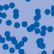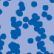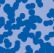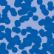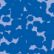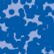## News

Florian Schweiger erhielt den Hausdorff-Gedächtnispreis 2021 der Fachgruppe Mathematik für die beste Dissertation. Er fertigte die Dissertation unter der Betreuung von Prof. Stefan Müller an. Unter anderen wurde Vanessa Ryborz mit einem Preis der Bonner Mathematischen Gesellschaft für ihre von Prof. Sergio Conti betreute Bachelorarbeit ausgezeichnet. (18.01.2022)

Prof. Dr. Sergio Albeverio has been elected into the Academia Europaea and the Accademia Nazionale dei Lincei (more; 02.12.2021).

Der SFB 1060 Die Mathematik der emergenten Effekte hat eine dritte Förderperiode erhalten. (26.11.20)

Prof. Dr. Andreas Eberle erhält den diesjährigen Lehrpreis der Universität Bonn. (22.07.2020)

Herr Dr. Richard Höfer erhielt den Hausdorff-Gedächtnispreis 2019 der Fachgruppe Mathematik für die beste Disseration. Betreut wurde die Arbeit von Prof. J. Velázquez (29.01.2020).

Contact

Managing Director: Prof. Dr. Massimiliano Gubinelli